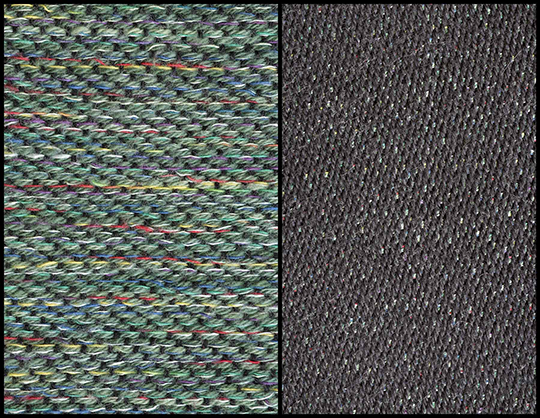# Satins and Sateens (Part I)

#### Marcy Petrini

May, 2017

In the current issue of Shuttle Spindle & Dyepot (Issue 190 Summer 2017), Penny Morgan in her President’s letter showed a beautiful fabric and briefly mentioned satin and sateen.

The question came up: how do you weave a satin? And how do you weave a sateen?

A satin is an unbalanced weave: one side is weft-dominant, sometimes referred to as the “sateen” side (left fabric below), the other side is warp-dominant, referred to as the “satin” side (right side below). Of course, once off the loom, this distinction doesn’t matter; by looking at the fabric below, there is no way of telling which side was up on the loom. But generally, for ease of treadling, we often lift the fewer shafts possible, thus weaving the sateen side up.From the fabric, we can see that the floats are not organized; they are interrupted or stitched down intermittently by a single warp thread; we start with a straight draw and stitch it by a step. There are a rules on how to obtain possible steps.

We need at least 5 shafts to weave a satin, for reasons that become clear when we look at the rules for the steps. And not all straight draws above 5 can be woven as satins; 6, for example, can’t. Here is why:

 Rules Example for 5-shaft satin No 6-shaft satin Start with a straight draw for n number of shafts, with n at least 5 1, 2, 3, 4, 5 1, 2, 3, 4, 5, 6 Do not use a step number of n because it wouldn’t allow any threading Step cannot be 5 Step cannot be 6 Do not use a step number of 1 or (n - 1) since it wouldn’t change the straight draw Step cannot be 1 or 4 Step cannot be 1 or 5 The step number cannot have a common factor (mathematically) with n 2 and 3 do not have a common factor with 5 2, 3 and 4 are factors of 6 The remaining integers can be used as steps Step of 2 or 3 are possible There are no step left!

Here is how we apply the steps, using as an example the 5-shaft satin and the two possible steps:

 Step of 2 Step of 3 1 1 1 + 2 = 3 1 + 3 = 4 3 + 2 = 5 4 + 3 = 7 – 5 = 2 5 + 2 = 7 – 5 = 2 2 + 3 = 5 2 + 2 = 4 5 + 3 = 8 – 5 = 3 4 + 2 = 6 – 5 = 1, the starting point 3 + 3 = 6 – 5 = 1, the starting point Threading: 1, 3, 5, 2, 4, repeat Threading: 1, 4, 2, 5, 3, repeat

It doesn’t matter which step we use, the fabrics are very similar, as shown in the following drawdown, black warp is a step of 2, blue warp a step of 3; the first half of the treadling produces the sateen side, the second half the satin side.Click here for the full-sized draft draft for this 5 shaft satin (a PDF will open in a new window).

Are steps for 7 and 8 shaft satins possible? Yes! Do you see why? And do you see why we cannot have a 4-shaft satin? It is also possible to thread a straight draw and apply the step to the treadling. How does that fabric differ?